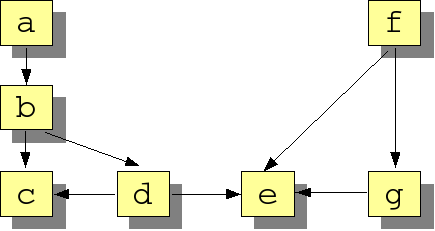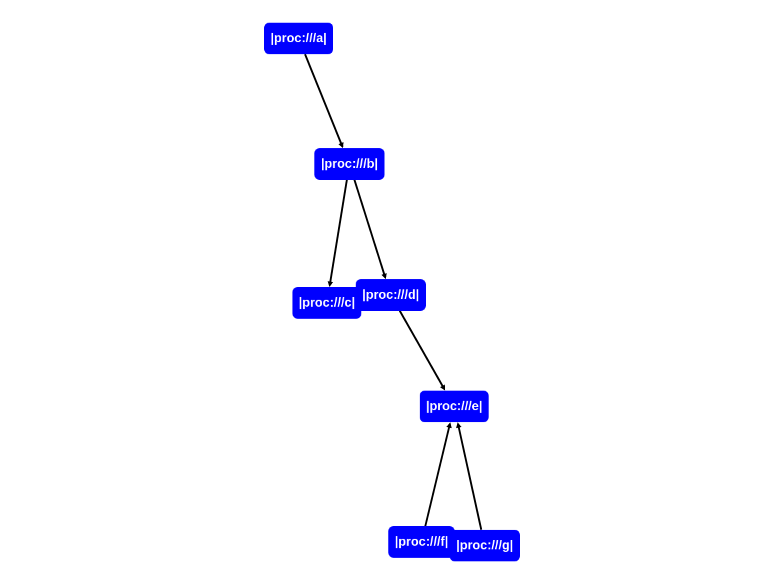# Call Analysis

rascal-0.33.7

#### Synopsis​

Analyzing the call structure of an application.

#### Description​

Suppose a mystery box ends up on your desk. When you open it, it contains a huge software system with several questions attached to it:

• How many procedure calls occur in this system?
• How many procedures does it contain?
• What are the entry points for this system, i.e., procedures that call others but are not called themselves?
• What are the leaves of this application, i.e., procedures that are called but do not make any calls themselves?
• Which procedures call each other indirectly?
• Which procedures are called directly or indirectly from each entry point?
• Which procedures are called from all entry points?

Let's see how these questions can be answered using Rascal.

#### Examples​

Consider the following call graph (a box represents a procedure and an arrow represents a call from one procedure to another procedure):``rascal>import Set;okrascal>import Relation;okrascal>import analysis::graphs::Graph;ok``

Rascal supports basic data types like integers and strings which are sufficient to formulate and answer the questions at hand. However, we can gain readability by introducing separately named types for the items we are describing. First, we introduce a new type `Proc` (an alias for locations) to denote procedures:

``rascal>alias Proc = loc;ok``

`loc` is the default data type used by Rascal programmers to encode "qualified names", i.e. these are the names invented for source code artifacts after they have been resolved by name and type analysis within their respective declaration scopes.

Next, we have to represent the call relation as a Rascal datatype, and the relation is the most appropriate for it. As preparation, we also import the libraries Set, Relation and Graph that will come in handy.

``rascal>rel[Proc, Proc] calls = {<|proc:///a|, |proc:///b|>, <|proc:///b|, |proc:///c|>, <|proc:///b|, |proc:///d|>, <|proc:///d|, |proc:///c|>, >>>>>>>                         <|proc:///d|, |proc:///e|>, <|proc:///f|, |proc:///e|>, <|proc:///f|, |proc:///g|>, <|proc:///g|, |proc:///e|>};rel[loc,loc]: {  <|proc:///a|,|proc:///b|>,  <|proc:///g|,|proc:///e|>,  <|proc:///b|,|proc:///c|>,  <|proc:///b|,|proc:///d|>,  <|proc:///d|,|proc:///c|>,  <|proc:///d|,|proc:///e|>,  <|proc:///f|,|proc:///e|>,  <|proc:///f|,|proc:///g|>}``

let's first visualize the calls relation to get an overview:

``rascal>import vis::Graphs;okrascal>graph(calls)``Now we are in a good position to start asking some questions.

How many calls occur in this system?

We use the function Size to determine the number of elements in a set or relation. Since each tuple in the `calls` relation represents a call between procedures, the number of tuples is equal to the number of calls.

``rascal>size(calls);int: 8``

How many procedures occur in this system?

This question is more subtle, since a procedure may call (or be called) by several others and the number of tuples is therefore not indicative. What we need are the set of procedures that occur (as first or second element) in any tuple. This is precisely what the function Carrier gives us:

``rascal>carrier(calls);set[loc]: {  |proc:///a|,  |proc:///b|,  |proc:///c|,  |proc:///d|,  |proc:///e|,  |proc:///f|,  |proc:///g|}``

and computing the number of procedures is now easy:

``rascal>size(carrier(calls));int: 7``

As an aside, functions Domain and Range do the same for the first, respectively, second element of the pairs in a relation:

``rascal>domain(calls);set[loc]: {  |proc:///a|,  |proc:///b|,  |proc:///d|,  |proc:///f|,  |proc:///g|}rascal>range(calls);set[loc]: {  |proc:///b|,  |proc:///c|,  |proc:///d|,  |proc:///e|,  |proc:///g|}``

What are the entry points for this system?

The next step in the analysis is to determine which entry points this application has, i.e., procedures which call others but are not called themselves. Entry points are useful since they define the external interface of a system and may also be used as guidance to split a system in parts. The top of a relation contains those left-hand sides of tuples in a relation that do not occur in any right-hand side. When a relation is viewed as a graph, its top corresponds to the root nodes of that graph. Similarly, the bottom of a relation corresponds to the leaf nodes of the graph. See the module called Graph for more details. Using this knowledge, the entry points can be computed by determining the top of the calls relation:

``rascal>top(calls);set[loc]: {  |proc:///a|,  |proc:///f|}``

What are the leaves of this application?

In a similar spirit, we can determine the leaves of this application, i.e., procedures that are being called but do not make any calls themselves:

``rascal>bottom(calls);set[loc]: {  |proc:///c|,  |proc:///e|}``

Which procedures call each other indirectly?

We can also determine the indirect calls between procedures, by taking the transitive closure of the calls relation, written as `calls+`. Observe that the transitive closure will contain both the direct and the indirect calls.

``rascal>closureCalls = calls+;rel[loc,loc]: {  <|proc:///g|,|proc:///e|>,  <|proc:///a|,|proc:///b|>,  <|proc:///a|,|proc:///c|>,  <|proc:///a|,|proc:///d|>,  <|proc:///a|,|proc:///e|>,  <|proc:///b|,|proc:///c|>,  <|proc:///b|,|proc:///d|>,  <|proc:///b|,|proc:///e|>,  <|proc:///d|,|proc:///c|>,  <|proc:///d|,|proc:///e|>,  <|proc:///f|,|proc:///e|>,  <|proc:///f|,|proc:///g|>}``

Which procedures are called directly or indirectly from each entry point?

We now know the entry points for this application (|proc:///a| and |proc:///f|) and the indirect call relations. Combining this information, we can determine which procedures are called from each entry point. This is done by indexing `closureCalls` with appropriate procedure name. The index operator yields all right-hand sides of tuples that have a given value as left-hand side. This gives the following:

``rascal>calledFromA = closureCalls[|proc:///a|];set[loc]: {  |proc:///b|,  |proc:///c|,  |proc:///d|,  |proc:///e|}rascal>calledFromF = closureCalls[|proc:///f|];set[loc]: {  |proc:///e|,  |proc:///g|}``

Which procedures are called from all entry points?

Finally, we can determine which procedures are called from both entry points by taking the intersection of the two sets `calledFromA` and `calledFromF`:

``rascal>calledFromA & calledFromF;set[loc]: {|proc:///e|}``

or if your prefer to write all of the above as a one-liner using a Reducer expression:

``rascal>(carrier(calls) | it & (calls+)[p] | p <- top(calls));set[loc]: {|proc:///e|}``

The reducer is initialized with all procedures (`carrier(calls)`) and iterates over all entry points (`p <- top(calls)`). At each iteration the current value of the reducer (`it`) is intersected (`&`) with the procedures called directly or indirectly from that entry point (`(calls+)[p]`).

#### Benefits​

• In small examples, the above results can be easily obtained by a visual inspection of the call graph. Such a visual inspection does not scale very well to large graphs and this makes the above form of analysis particularly suited for studying large systems.

#### Pitfalls​

• We discuss call analysis in an intentionally, simplistic fashion that does not take into account how the call relation is extracted from actual source code. The above principles are, however, applicable to real cases as well.